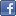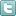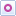EE Times-India > EDA/IP

EDA/IP# Boost fault coverage for random-pattern-resistant design

Posted: 14 Dec 2015Print Version

Keywords:LogicBIST  LBIST  random resistant fault analysis  fault detection  Pseudo Random Pattern Generator

Designs with LogicBIST exhibit random pattern resistance because of the random nature of LBIST vectors, thus leading to low fault coverage. To handle this, we insert test points with the help of random resistant fault analysis (RRFA). The computation of the fault detection capability of a design with LBIST is done with the help of fault simulation, which gives an estimate of "quality of test". We discuss these in more detail below, along with techniques to increase fault detection in LBIST designs.

Fault targeting with LogicBIST
Test through LBIST is a pseudo random test unlike the production scan test which is more deterministic test. The scan vectors in LBIST are generated by a Pseudo Random Pattern Generator (PRPG) which generates pseudo random sequences. Whereas in the case of production scan testing the scan vectors are deterministically fed through the scan inputs through the Automatic Test Equipment (ATE).

Due to the random nature of LBIST test it is not always possible to test particular faults because of no direct control on what sequence of scan inputs is being shifted in the design. Problems arise when LBIST is implemented on designs which are combinational intense or have large combinational paths between the registers. These designs may become resistant to random patterns which means the probability of controlling some nodes randomly to a 0 or 1 value, or the probability of observing some nodes to a scan-register is low, assuming random and equally probable inputs being fed to the design.

Taking example of an AND gate in figure 1 we calculate the probability of controlling the output of the gate to a value '1'. The diagram below shows the probability of each node getting a value of '1' or '0'. Format P(1) / P(0).Figure 1: Controllability for 2 input and gate.

Figure 2 the probability of getting '0' or '1' value at the different nodes when the combinational depth is increased by one. The probability of getting '1' at the output of this combinational block is 1/8. However this is still a very simple combinational block, and we see complex blocks in real design. So, as the combinational depth increases the ability to control the nodes to a particular value decreases.Figure 2: Controllability for 2 depth combo logic.

Such designs show resistance to random pattern when tested with LBIST and may lead to low fault coverage. To handle such scenarios we often insert points to improve the design's testability. Test points can be categorised in two types: Control points and observe points.

 Related Articles Editor's Choice
Comment on "Boost fault coverage for random-patt..."

Top Ranked Articles

WebinarsVisit Asia Webinars to learn about the latest in technology and get practical design tips.

Search EE Times India
Services

Go to topConnect on FacebookFollow us on TwitterFollow us on Orkut
﻿

Back to Top